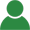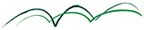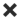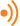ErrorHome Courses Course Calendar Teaching Team About Us Resources Contact

Can't travel? Don't want to travel? LFS Livebrings the class to you!

• Live interactive training from world renowned practitioners in the comfort of your own home
• Real classroom experience without the inconvenience of travel
• World class teaching from the comfort of your preferred location

Course Calendar

# Maths Refresher for Finance

## Day One

### Numbers, Algebra and Functions

• Linear, quadratic and other polynomial functions
• Numerical methods and the Taylor series
• Role of the exponential function in Finance

Workshops:

• Bonds and Bond Yields
• Modelling Yield Curves with polynomials and splines

### Differentials and Calculus

• Gradients, rates of change and differential calculus
• Partial Differentials and PDEs
• Definite and indefinite integrals

Workshops:

• Techniques in calculus
• Bond Prices, Interest Rates and Duration
• Role of the Diffusion Equation PDE in Finance

### Matrix Algebra

• Matrix rules and operations
• Cofactors and Determinants
• The Portfolio Matrix

Workshops:

• Simultaneous equations and solutions
• Payoff Matrices
• Portfolio risk & return and the Variance-Covariance matrix

## Day Two

### Probability and Sets

• Sets, diagrams, notation and probability laws
• Probability Trees and Discrete Probability Distributions
• Hypothesis or Significance Testing

Workshops:

• Probability Calculations
• Expected Monetary Value
• Significance Testing with Trading Data

### Distributions and Stochastic Processes

• Real-world Distributions and Descriptive Statistics
• Theoretical probability distributions – Binomial, Normal and others
• Stochastic approaches to Financial Data
• Random walks and the Wiener Process

Workshops:

• Descriptive Statistics and the FTSE
• Random variables and Random walks
• Stochastic Equity Returns Modelling

### Forecasting and Regression

• Time-series Approaches
• Correlation, Variance and Covariance
• Linear and multiple regression
• Real data and cautions in forecasting

Workshops:

• Simple linear regression
• Multiple regression forecasting models

### Further Advanced Workshop Excel Examples:

• Simple and Log returns, Volatility and VaR
• Log returns, Alpha, Beta and the Security Characteristic Line
• Modern Portfolio Theory and CAPM
• Modelling Bond Default with Probability Transition Matrices